# Resistor Color Code Calculator

Created by Luis Hoyos
Last updated: Nov 16, 2022

With this resistor color code calculator, you can calculate the electrical resistance of your resistor by inputting the colors that appear on it. Just choose the resistor type, select the colors, and the tool will display the resistance, tolerance, and range on the spot. You can calculate resistor color codes of 4 bands, 5 bands, or 6 bands, containing any color.

Keep reading this article to learn what resistor colors mean, how to calculate the resistance manually, and the corresponding color codes of a 10k resistor. Also, visit the Ohm's law calculator to find voltage or current based on the resistance

## What do the color bands mean?

TLDR: Resistor color codes represent different numbers or digits that we input in a formula to calculate the electrical resistance of the resistor.

#### First 2-3 bands

The colors of the first 2-3 bands are related to a series of digits in the following way:

color name

digit

color

color name

digit

color

black

$0$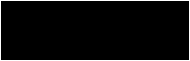green

$5$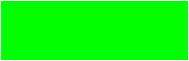brown

$1$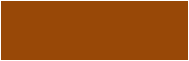blue

$6$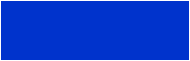red

$2$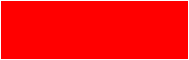violet

$7$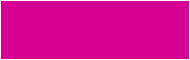orange

$3$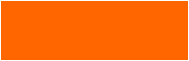gray

$8$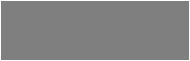yellow

$4$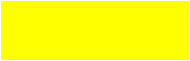white

$9$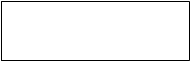• For a four bands resistor, the above table describes the first two bands; and
• For the five and six bands resistors, the previous table describes the first three bands.

#### Multiplier band

The colors of the multiplier band have the following meaning:

color name

multiply

color

color name

digit

color

black

$\times1\ \mathrm{Ω}$blue

$\times1\ \mathrm{MΩ}$brown

$\times10\ \mathrm{Ω}$violet

$\times10\ \mathrm{MΩ}$red

$\times100\ \mathrm{Ω}$gray

$\times100\ \mathrm{MΩ}$orange

$\times1\ \mathrm{kΩ}$white

$\times1\ \mathrm{Ω}$yellow

$\times10\ \mathrm{kΩ}$gold

$\times10\ \mathrm{kΩ}$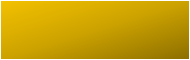green

$\times0.1\ \mathrm{Ω}$silver

$\times0.01\ \mathrm{Ω}$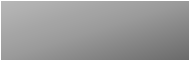• For a four bands resistor, the previous table describes the third band; and
• For the five and six bands resistors, the previous table describes the fourth band.

#### Tolerance band

The tolerance band is related to the error or uncertainty of the resistance value of the resistor.

color name

multiply

color

color name

digit

color

brown

$\pm1\%$violet

$\pm0.1\%$red

$\pm2\%$gray

$\pm0.05\%$green

$\pm0.5\%$gold

$\pm5\%$blue

$\pm0.25\%$silver

$\pm10\%$• For a four bands resistor, the previous table describes the fourth band; and
• For the five and six bands resistors, the previous table describes the fifth band.

#### Temperature coefficient band

The temperature coefficient band defines how resistance changes as a function of the ambient temperature, using the temperature coefficient of resistance (TCR).

TCT expresses this change using parts per million per celsius degree ($\mathrm{ppm/\degree C}$)

• ppm is a way to express (very small) fractions and, therefore, percentages. For example, five parts per million represent a 0.0005% change: $5 \text{ ppm} = \frac{5}{1000000} = 0.000005 = 0.0005\%$
• If $\text{TCR} = 5 \mathrm{\ ppm /\degree C}$, it means that per every celsius degree change in ambient temperature, there will be a 0.0005% change in the actual value of the resistance.

color name

TCR [$\boldsymbol{\mathrm{ppm/\degree C}}$]

color

color name

digit

brown

$100$yellow

$25$red

$50$blue

$10$orange

$15$violet

$5$The temperature coefficient band only appears in six bands resistors.

## Manual calculation of resistor color code

• For the 4-band resistor color code, we calculate the resistance we the following formula:
$R = \mathrm{band}_3 \times ((10\times\mathrm{band}_1)+\mathrm{band}_2)\pm\mathrm{band}_4$
• For the 5-band resistor, we use the following formula:
$R = \mathrm{band}_4\times ((100\times\mathrm{band}_1) + (10 \times \mathrm{band}_2) +\mathrm{band}_3) \pm \mathrm{band}_5$
• For the 6-band resistor, we use the same formula as for the 5-band resistor, adding the TCR to the result.

## What are the color codes of a 10k resistor?

One of the most common electronic devices is the 10k ohm resistor, and its color codes depend on whether it has four or five bands.

• For a four bands resistor, the color codes of the 10k ohm resistor are brown, black, orange, + any color band.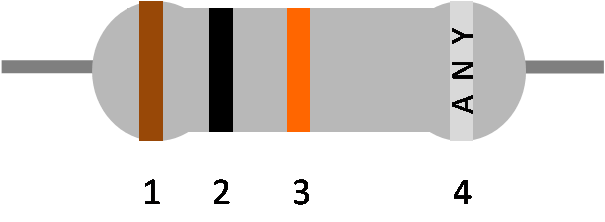• For a five bands resistor, the color codes of the 10k ohm resistor are brown, black, black, red, + any color band.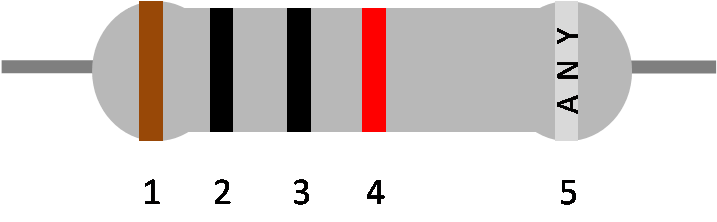• For a six bands resistor, the color codes of the 10k ohm resistor are the same as the five bands resistors (brown, black, black, red + any color band) plus the temperature coefficient band.

🔎 Now that you know how to read your resistor, it's time to design some circuits! We have a great collection of tools to help you do so:

Luis Hoyos
Number of bands
four
Bands colors
Band 1
Select...
Band 2
Select...
Multiplier
Select...
Tolerance
Select...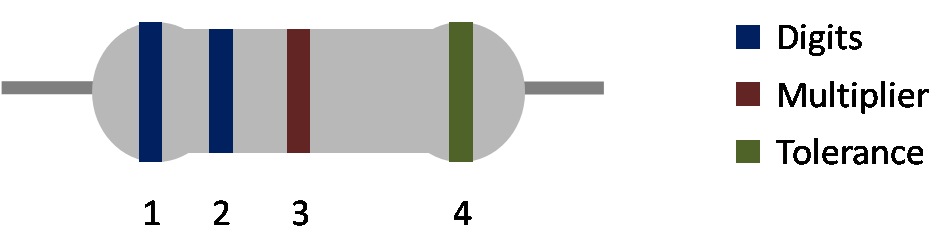People also viewed…

### Inductive reactance

Find the inductive reactance of an inductor with our inductive reactance calculator!

### Resistor wattage

Obtain the wattage of any resistor with our resistor wattage calculator!